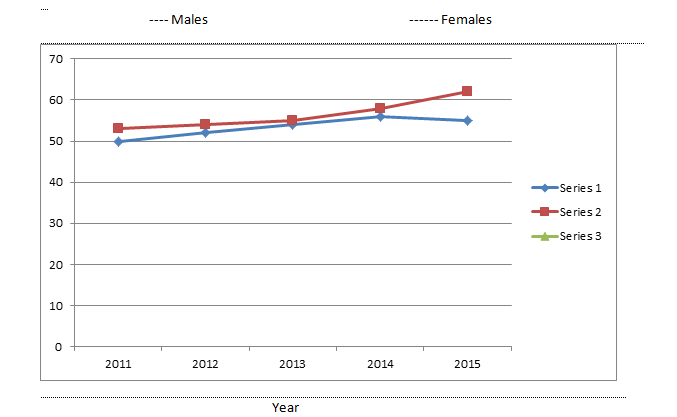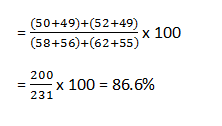New Students Offer - Use Code HELLO

# RBI Assistant: Important Data Interpretation (Set 1)

Direction (1-5): Study the bar graph and line graph carefully to answer the questions given below.
The bar graph shows the number of males and females (in thousand) in town X during the given years.The line graph shows the number of males and females (in thousand) in town Y during the given years.1. What is the ratio of the average number of males in town X to the average of males in town Y for the given period?
1. 269:282
2. 265:281
3. 265:283
4. 265:282
5. Other than the given options

2. In which of the following years, is the percentage increase or decrease in the number of females for town Y the minimum?
1. 2015
2. 2014
3. 2012
4. 2013
5. Both 1) and 2)

3. The population o town X in 2011 and 2012 together is approximately what per cent of the population of town Y in 2014 and 2015 together?
1. Other than the given options
2. 81.6%
3. 89.6%
4. 84.5%
5. 86.6%

4. Find the number of years in which the number if females in town X and Y are less their respective average numbers.
1. One, Two
2. Two, Two
3. None
4. Three, Two
5. Other than the given options

5. In which of the following pairs of years in the difference in the number of males and females the maximum for town Y and minimum for town Y and minimum for town X respectively?
1. 2011 and 2014
2. 2015 and 2011
3. 2015 and 2014
4. 2013 and 2015
5. Other than the given options

Take 200 Data Interpretation Practice Sets here

1. 4; Required ratio2. 1; The percentage increase or decrease in the number of females for town Y are as under;Hence, minimum is in 2015.

3. 5; Required percentage4. 2; Average number of females for town X = 51000
Average number of females for town Y = 53400
So, 2011 and 2012 are two desired years for town X. Also, 2011 and 2012 are two desired years for town Y.

5. 3; Difference between the population of males and females;

 2011 2012 2013 2014 2015 X 1000 3000 3000 0 3000 Y 3000 2000 1000 2000 7000

∴ Desired pair is 2015 and 2014.